# SAT Math Multiple Choice Question 163: Answer and Explanation

### Test Information

Question: 163

13. For which of the following values of w does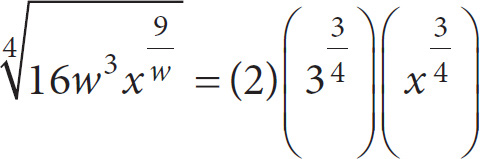?

• A. 2
• B. 3
• C. 4
• D. 6

B Taking the 4th root of a number is the same as taking the number to the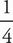power. Therefore, the equation can be rewritten as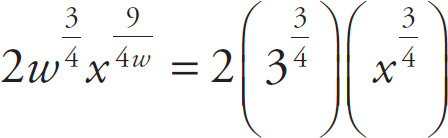. Divide both sides by 2 to get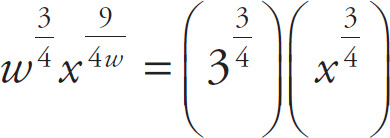. Therefore, in the equation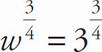and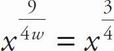, so w = 3. The correct answer is (B).